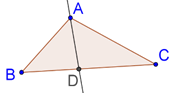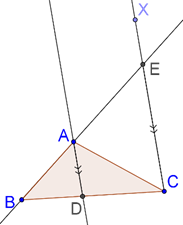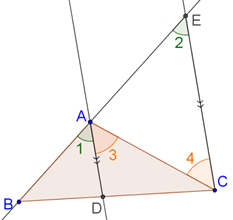# Angle Bisector Theorem

Go back to  'Similar-Triangles'

An important consequence of the BPT is the following theorem.

Angle Bisector Theorem: In a triangle, the angle bisector of any angle will divide the opposite side in the ratio of the sides containing the angle.

Consider the figure below, in which AD is the bisector of $$\angle A$$. Our theorem tells us that BD:DC = AB:AC.Proof: Draw a ray CX parallel to AD, and extend BA to intersect this ray in E:By the BPT, BD:DC = BA:AE. Now, we will prove that AE = EC. For that, consider the figure above once again, with the angles marked, as shown below:We have:

• $$\angle1$$ = $$\angle 2$$ (corresponding angles)

• $$\angle 3$$ = $$\angle 4$$ (alternate interior angles)

But AD is the bisector of $$\angle BAC$$, which means that $$\angle 1$$ = $$\angle 3$$. Thus, $$\angle 2$$ = $$\angle 4$$, which further implies that AC = AE. Finally, this means that:

$\frac{{BD}}{{DC}} = \frac{{BA}}{{AE}} = \frac{{BA}}{{AC}}$

The angle bisector AD thus divides the opposite side BC in the ratio of the sides (AB and AC) containing that angle.

Learn math from the experts and clarify doubts instantly

• Instant doubt clearing (live one on one)
• Learn from India’s best math teachers
• Completely personalized curriculum